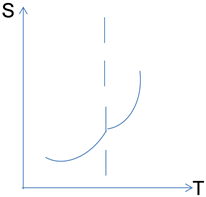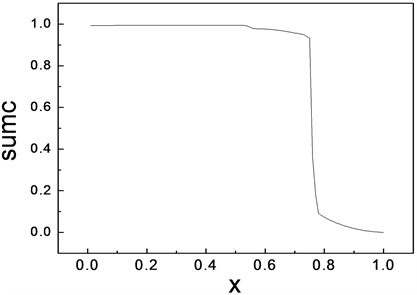﻿ 关于二级相变中的熵变分析

# 关于二级相变中的熵变分析The Analysis of the Entropychange in Second-Order Phase Transition

Abstract: The entropy in the second-order phase transition of matter is studied in this paper. Firstly, the characteristics of second order phase transition and entropy in second order phase transition are introduced. Then, the von Neumann entropy is calculated and simulated with the spin chain model to reveal the mechanism of the second-order phase transition. It is significant to understand deeply the internal origin of the second-order phase transition and the characteristics of the second-order phase transition.

1. 引言

2. 相关理论

2.1. 二级相变特征

${\left(\frac{\partial {\mu }_{1}}{\partial T}\right)}_{P}={\left(\frac{\partial {\mu }_{2}}{\partial T}\right)}_{P},{S}_{1}={S}_{2}$ (1)

${\left(\frac{\partial {\mu }_{1}}{\partial P}\right)}_{T}={\left(\frac{\partial {\mu }_{2}}{\partial P}\right)}_{T},{V}_{1}={V}_{2}$ (2)

$\frac{{\partial }^{2}{\mu }_{1}}{\partial {T}^{2}}\ne \frac{{\partial }^{2}{\mu }_{1}}{\partial {T}^{2}},\frac{{\partial }^{2}{\mu }_{1}}{\partial T\partial P}\ne \frac{{\partial }^{2}{\mu }_{1}}{\partial T\partial P},\frac{{\partial }^{2}{\mu }_{1}}{\partial {P}^{2}}\ne \frac{{\partial }^{2}{\mu }_{1}}{\partial {P}^{2}}$ (3)

${C}_{P}=T\cdot {\left(\frac{\partial S}{\partial T}\right)}_{P}=-\frac{{\partial }^{2}{\mu }_{1}}{\partial {T}^{2}}$ (4)

$\alpha =\frac{1}{V}\cdot {\left(\frac{\partial V}{\partial T}\right)}_{P}=\frac{1}{V}\cdot \frac{{\partial }^{2}{\mu }_{1}}{\partial T\partial P}$ (5)

${K}_{T}=-\frac{1}{V}\cdot {\left(\frac{\partial V}{\partial P}\right)}_{T}=\frac{1}{V}\cdot \frac{{\partial }^{2}{\mu }_{1}}{\partial {P}^{2}}$ (6)

2.2. 二级相变中的熵情况Figure 1. The relationship between the entropy and temperature in the second-order phase transition

$\text{d}G={\left(\frac{\partial G}{\partial T}\right)}_{P}\text{d}T+{\left(\frac{\partial G}{\partial P}\right)}_{T}\text{d}P$ (7)

$\text{d}G=-S\text{d}T+V\text{d}P$ (8)

(8)式对比(7)式得： $-S={\left(\frac{\partial G}{\partial T}\right)}_{P},V={\left(\frac{\partial G}{\partial P}\right)}_{T}$

${G}_{0}\left(T,P,0\right)-{G}_{n}\left(T,P,0\right)=\frac{1}{2}{\mu }_{0}V{H}_{e}^{2}$ (10)

${S}_{0}\left(T,P,0\right)-{S}_{t}\left(T,P,0\right)=-{\mu }_{0}V{H}_{e}\frac{\text{d}{H}_{e}}{\text{d}T}$ (11)

${C}_{0}-{C}_{t}=-{\mu }_{0}VT\left[{H}_{e}\frac{{\text{d}}^{2}{H}_{e}}{\text{d}{T}^{2}}+{\left(\frac{\text{d}{H}_{e}}{\text{d}T}\right)}^{2}\right]$ (12)

${T}_{0}={T}_{e}$ 时，在临界温度时热容没有变化。

$Q=T\left({S}_{0}-{S}_{t}\right)=-{\mu }_{0}TV{H}_{e}\frac{\text{d}{H}_{e}}{\text{d}T}$ (13)

${T}_{0}<{T}_{e}$ 时，由超导向正常相变过程发生吸热，正常向超导相变过程发生放热，这是一级相变。但当 $T\to 0$$Q=0$，无相变潜热，但有热容变化，因此在 ${T}_{0}={T}_{e}$ 时，无相变潜热，有热容变化，属于二级相变。

3. 使用自旋链模型对二级相变中的熵情况进行模拟分析

3.1. 一维自旋链模型的哈密顿量

3.2. N格点自旋链模型的von Neumann熵

$S=-\frac{1}{N}\underset{i=1}{\overset{N}{\sum }}\left[{C}_{i}^{2}{\mathrm{log}}_{2}\left({C}_{i}^{2}\right)+\left(1-{C}_{i}^{2}\right){\mathrm{log}}_{2}\left(1-{C}_{i}^{2}\right)\right]$ (这里i的取值从1到N，其中 ${C}_{i}$ 原本的意义为基态波函

3.3. 计算结果Table 1. The table of the change of the von Neumann entropy (sumc) of the ground state along with the change of the parameter x of [11, 5] in the spin chain modelFigure 2. The change of the ground state entanglement (sumc) of the spin-chain XXZ Heisenberg model along with the change of parameter x in one-dimensional

3.4. 分析与结论

NOTES

*通讯作者。

 秦允豪. 热学[M]. 第四版. 北京: 高等教育出版社, 2018: 168-183.

 汪志成. 热力学•统计物理[M]. 第三版. 北京: 高等教育出版社, 1984.

 李越, 任恒峰, 王清亮. 离子晶体中熵的性质[J]. 商丘师范学院学报, 2021, 37(3): 29-31.

 王冬梅, 谢鑫. 基于信息熵的自适应高斯金字塔的LSD算法改进[J]. 吉林大学学报, 2020, 38(6): 647-655.

 张蕾. 斯格明子相关的螺旋磁有序体系的临界行为[J]. 物理学报, 2018, 6(13): 137501-137521.

 徐斌涛. 超导体相变的热力学行为[J]. 上海海运学院学报, 1994(5): 47-51.

 韩文娟, 周勋. 对一维XXZ海森堡开链模型链基态纠缠度计算的分析[J]. 贵州大学学报(自然科学版), 2010, 24(3): 25-28.

 方贺男, 陶志阔. 基于朗道二级相变理论的铁单晶磁结构分析[J]. 南京邮电大学学报, 2014, 34(1): 133-136.

 韩文娟, 强睿. 关于二级相变中热力学物理量突变的分析与自旋链模拟[J]. 量子光学学报, 2017, 23(2): 105-110.

Top